# Overview

$${\tt BigVAR}$$ is the companion R package to the papers "VARX-L: Structured Regularization for Large Vector Autoregression with Exogenous Variables" (Joint with David Matteson and Jacob Bien) and "High Dimensional Forecasting via Interpretable Vector Autoregression (HLag)" (Joint with Ines Wilms, David Matteson, and Jacob Bien).

$${\tt BigVAR}$$ allows for the simultaneous estimation and forecasting of high-dimensional time series by applying structured penalties to the standard vector autoregression (VAR) and vector autoregression with exogenous variables (VARX) frameworks. This is useful in many applications which make use of time-dependent data, such as macroeconomics, finance, and internet traffic, as the conventional VAR and VARX are heavily overparameterized. In addition, as stated in Ghysels and Marcellino (2018), VARs with "large enough" lag order can adequately approximate VARMA models.

Our package adapts solution methods from the regularization literature to a multivariate time series setting, allowing for the computationally efficient estimation of high-dimensional VAR and VARX models.

We also allow for least squares refitting based on the nonzero support selected by our procedures as well as the ability to incorporate mild non-stationarity by shrinking toward a vector random walk. For more information on these extensions, we refer you to our papers W. Nicholson, Matteson, and Bien (2017b) and Nicholson et al. (2020).

This vignette presents a brief formal overview of our notation, the models contained in $${\tt BigVAR}$$, and the functionality of the package. For an interactive tutorial see the shiny app. Any questions or feature requests with regard to $${\tt BigVAR}$$ can be addressed to wbn8@cornell.edu. If you have basic questions about VAR or multivariate time series in general, we recommend consulting Lütkepohl (2005).

Notation and Methodology provides an overview of the VARX model as well as the $${\tt BigVAR}$$ framework. Our penalty structures are described in VARX-L and HLAG. Empirical penalty parameter selection procedures are discussed in Penalty Parameter Selection and N-fold cross validation. Package specific syntax is detailed in BigVAR Details. Finally, example applications and extensions of $${\tt BigVAR}$$ are provided in Selecting a Structure and Impulse Response Functions.

## Installation

The stable version of $${\tt BigVAR}$$ is available on cran. The developmental release can be installed from github using the following command:

install_github("wbnicholson/BigVAR/BigVAR")

## Quick Start

In this section, we provide a basic overview of the capabilities of $${\tt BigVAR}$$. Further sections will provide elaboration as to the full functionality of $${\tt BigVAR}$$.

$$\mathbf{Y}$$, a simulated multivariate time series of dimension $$100\times 3$$ is included with $${\tt BigVAR}$$ and is used throughout this vignette (details as to its construction are provided in Example Data). It can be accessed by calling:

library(BigVAR)
data(Y)

In order to forecast $$\hat{y}_{t+1}$$ using a vector autoregression with a lasso penalty $$\lambda=1$$ and maximum lag order of 2, one can simply run

# 3 x 7 coefficient matrix
B = BigVAR.fit(Y,struct='Basic',p=2,lambda=1)[,,1]
# construct 7 x 99 lag matrix of Y
Z = VARXLagCons(Y,p=2,oos=TRUE)Z # obtain out of sample forecasts yhat = B%*%Z[,ncol(Z),drop=F] Some potential use cases of $${\tt BigVAR.fit}$$ are elaborated upon in Extensions. More sophisticated analysis requires the construction of an object of class $${\tt BigVAR}$$ as described in constructModel. ## Notation and Methodology Let $$\{ \mathbf{y_t}\}_{t = 1}^T$$ denote a $$k$$ dimensional vector time series and $$\{\mathbf{x}_t\}_{t=1}^{T}$$ denote an $$m$$-dimensional unmodeled series. A vector autoregression with exogenous variables of order (p,s) , VARX$$_{k,m}$$($$p,s$$), can be expressed as \begin{align} \label{VAR1} \mathbf{y}_t=\mathbf{\nu}+\sum_{\ell=1}^p\mathbf{\Phi}^{(\ell)}\mathbf{y}_{t-\ell}+\sum_{j=1}^s \mathbf{\beta}^{(j)}\mathbf{x}_{t-j}+\mathbf{u}_t \; \text{ for } \;t=1,\ldots,T, \end{align} in which $$\mathbf{\nu}$$ denotes a $$k\times 1$$ intercept vector, each $$\mathbf{\Phi}^{(\ell)}$$ represents a $$k\times k$$ endogenous (modeled) coefficient matrix, each $$\mathbf{\beta}^{(j)}$$ represents a $$k\times m$$ exogenous (unmodeled) coefficient matrix, and $$\mathbf{u}_t\stackrel{\text{wn}}{\sim}(\mathbf{0},\mathbf{\Sigma}_u)$$. Note that the VAR is a special case of Equation $$\ref{VAR1}$$ in which the second summation ($$\sum_{j=1}^s \mathbf{\beta}^{(j)}\mathbf{x}_{t-j}$$) is not included. ### Example Sparsity Patterns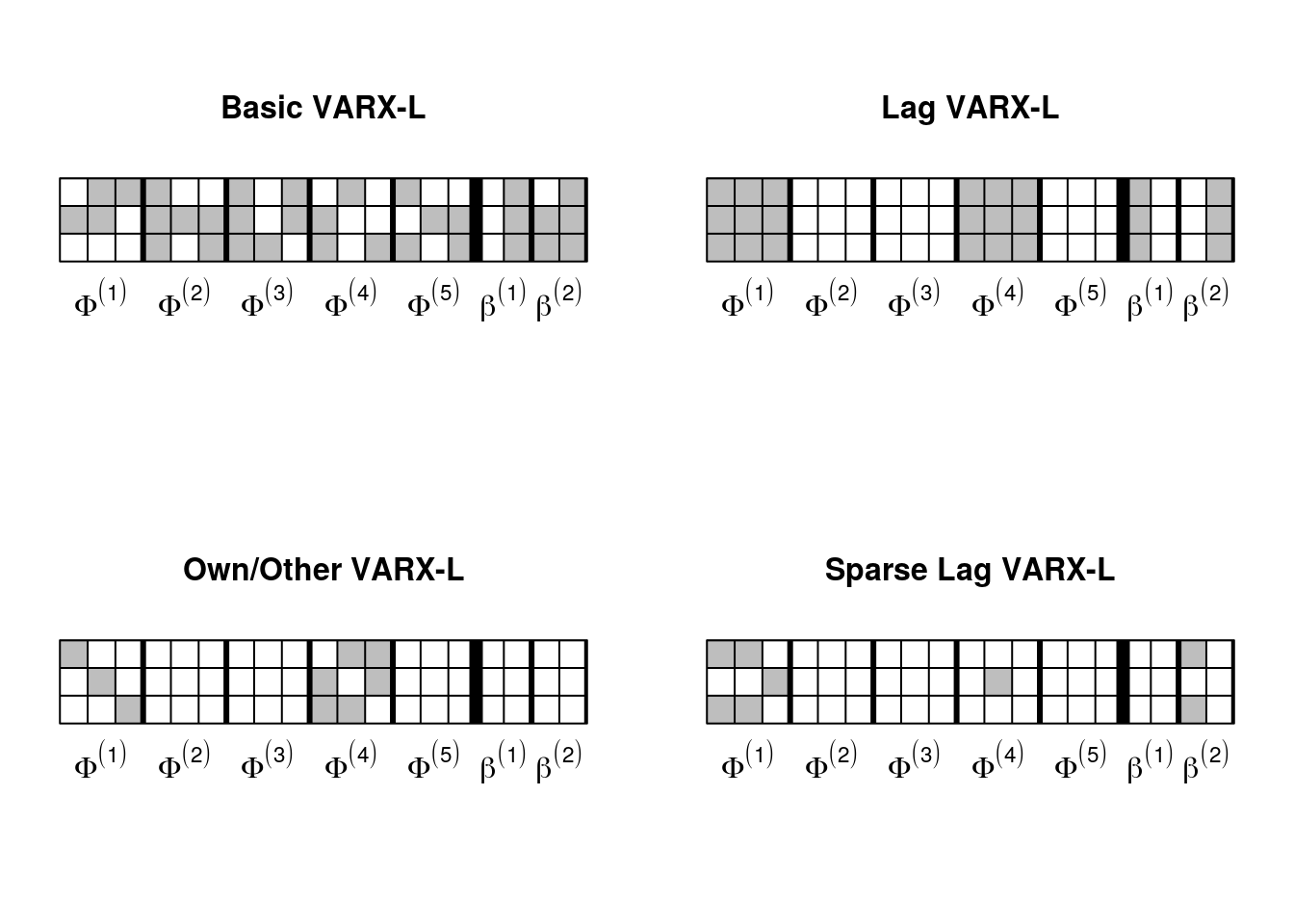1. The Basic VARX-L will zero individual elements in $$[\mathbf{\Phi},\mathbf{\beta}]$$ 2. The Lag VARX-L assigns a group lasso penalty by lag coefficient matrix $$\mathbf{\Phi}$$. Each column in the exogenous coefficient matrices $$\mathbf{\beta}$$ is assigned to its own group. 3. The Own/Other VARX-L assigns separate group lasso penalties to own lags ($$\text{diag}(\mathbf{\Phi}^{(\ell)})$$ and other lags $$(\mathbf{\Phi}^{(\ell)^{-}})$$. It applies the same penalty as the Lag VARX-L to the exogenous structure. 4. The Sparse Lag and Sparse Own/Other VARX-L extend the aforementioned structures to allow for within-group sparsity. We recently added an elastic net penalty (BasicEN) which combines the Basic VARX-L with a ridge penalty. ### VARX-L $$\tt BigVAR$$ can be used to apply the following penalties to the VARX (Equation $$\ref{VAR1}$$): Model Structure BigVAR Name Penalty Solution Algorithm Basic $$\tt{Basic}$$ $$\lambda\|[\mathbf{\Phi},\mathbf{\beta}]\|_1$$ Coordinate Descent Basic-Elastic Net $$\tt{BasicEN}$$ $$\lambda\big(\alpha\|[\mathbf{\Phi},\mathbf{\beta}]\|_1+(1-\alpha)\|[\mathbf{\Phi},\mathbf{\beta}]\|_2^2\big)$$ Coordinate Descent Lag VARX-L $$\tt{Lag}$$ $$\lambda\big(\sum_{\ell=1}^p\|\mathbf{\Phi}_\ell\|_F+\sqrt{k}\sum_{j=1}^s\sum_{i=1}^m\|\beta_{\cdot,i}^{(j)}\|_F \big)$$ Block Coordinate Descent Own/Other VARX-L $$\tt{OwnOther}$$ $$\lambda(\rho_1\sum_{\ell=1}^p \|\text{diag}(\mathbf{\Phi}^{(\ell)})\|_F+\gamma_1\sum_{\ell=1}^p\|\mathbf{\Phi}^{(\ell^{-})}\|_F+\sqrt{k}\sum_{j=1}^s\sum_{i=1}^m\|\beta_{\cdot,i}^{(j)}\|_F)$$ Block Coordinate Descent Sparse Lag VARX-L $$\tt{SparseLag}$$ $$(1-\alpha)\sqrt{k^2}\sum_{\ell=1}^p\|\mathbf{\Phi}^{(\ell)}\|_F+\alpha\|\mathbf{\Phi}\|_1 (1-\alpha)\sqrt{k}\sum_{j=1}^s\sum_{i=1}^m\|\beta_{\cdot,i}^{(j)}\|_F +\alpha\|\beta\|_1$$ Generalized Gradient Descent Own/Other Sparse VARX-L $$\tt{SparseOwnOther}$$ $$\lambda(1-\alpha)[\rho_1\sum_{\ell=1}^p \|\text{diag}(\mathbf{\Phi}^{(\ell)})\|_F+\gamma_1\sum_{\ell=1}^p\|\mathbf{\Phi}^{(\ell)^{-}}\|_F]+\alpha\lambda\|\mathbf{\Phi}\|{_1}$$ Generalized Gradient Descent $$\lambda>0$$ is a penalty parameter that can be selected via a validation procedure or chosen by the user; larger values of $$\lambda$$ encourage a greater degree of sparsity. $$0\leq \alpha\leq 1$$ is an additional penalty parameter set by default to $$\frac{1}{k+1}$$ to control within-group sparsity in the sparse group setting or the trade-off between the ridge and lasso penalty in the elastic net setting. We allow for $$\alpha$$ to be estimated empirically with the option $${\tt dual=TRUE}$$ in the function $${\tt constructModel}$$. $$\rho_1=\sqrt{k}$$ and $$\gamma_1=\sqrt{k(k-1)}$$ are fixed weights accounting for the cardinality of each group. ### HLAG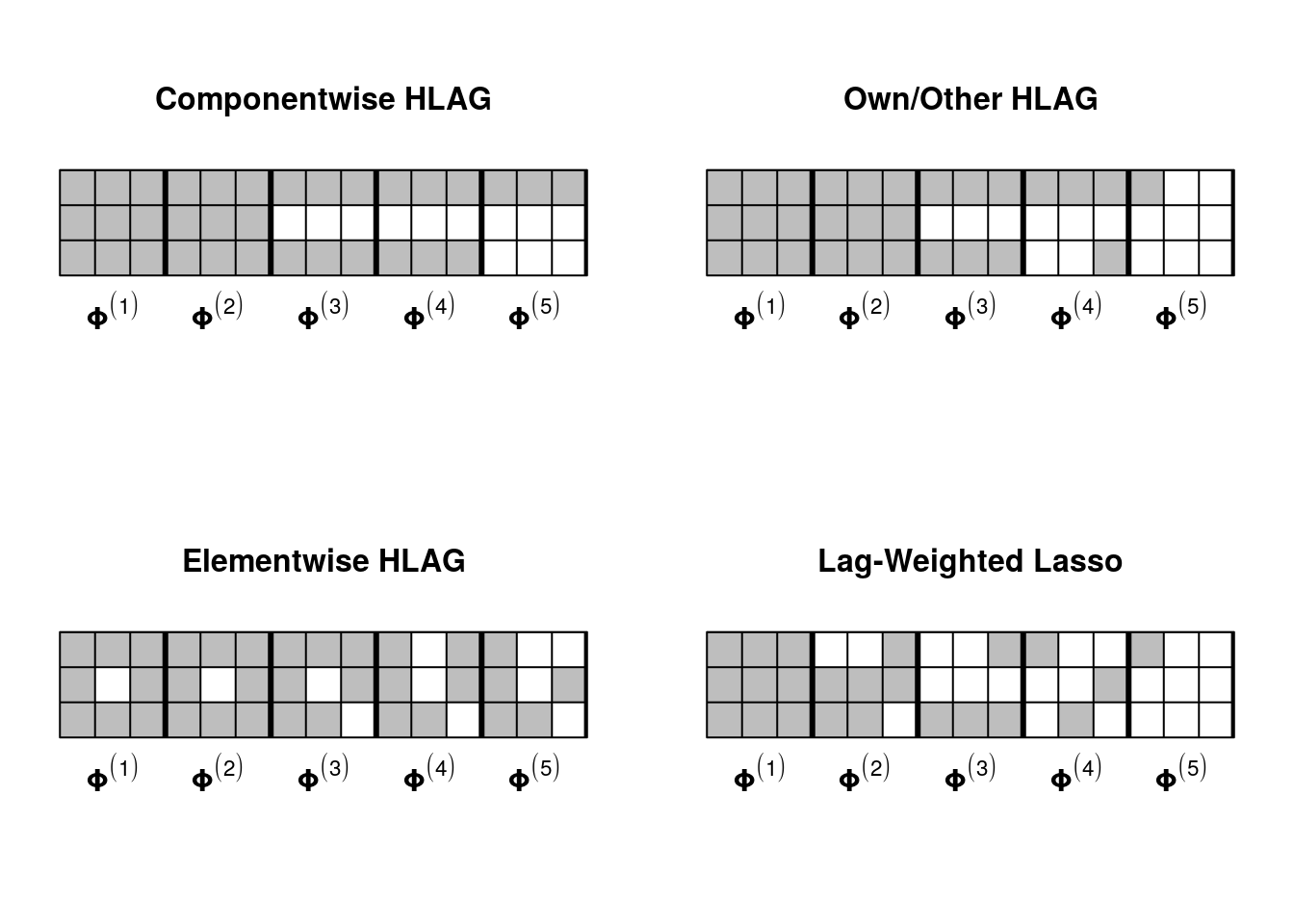Model Structure BigVAR Name Penalty Componentwise $$\tt{HLAGC}$$ $$\sum_{i=1}^k\sum_{\ell=1}^p\|\mathbf{\Phi}_i^{(\ell:p)}\|_2.$$ Own/Other $$\tt{HLAGOO}$$ $$\sum_{i=1}^k\sum_{\ell=1}^p\left[\|\mathbf{\Phi}_{i}^{(\ell:p)}\|_2+\|(\mathbf{\Phi}_{i,-i}^{(\ell)}, \mathbf{\Phi}_{i}^{([\ell+1]:p)})\|_2\right]$$. Elementwise $$\tt{HLAGELEM}$$ $$\sum_{i=1}^k\sum_{j=1}^k\sum_{\ell=1}^p\|\mathbf{\Phi}_{ij}^{(\ell:p)}\|_2$$. Lag-weighted Lasso $$\tt{Tapered}$$ $$\sum_{\ell=1}^p\ell^{\gamma}\|\mathbf{\Phi}^{(\ell)}\|_1$$. We additionally incorporate several VAR-specific penalties that directly address lag order selection. In addition to returning sparse solutions, our $$\text{HLAG}_k(p)$$ procedures induce regularization toward models with low maximum lag order. To allow for greater flexibility, instead of imposing a single, universal lag order (as information criterion minimization based approaches tend to do), we allow it to vary across marginal models (i.e. the rows of the coefficient matrix $$\mathbf{\Phi}=[\mathbf{\Phi}^{(1)},\dots,\mathbf{\Phi}^{(p)}]$$). $$\tt{BigVAR}$$ includes three HLAG models as well as the Lag-weighted Lasso, which incorporates a lasso penalty that increases geometrically as the lag order increases. This penalty does not directly address lag order but it encourages a greater degree of sparsity at more distant lags (as controlled by the additional penalty parameter $$\gamma \in (0,1)$$. The componentwise HLAG embeds a conventional lag order selection penalty into the hierarchical group lasso; the maximum lag order can vary across series, but within a series all components have the same maximum lag. The Own/Other HLAG adds another layer of lag order: within a lag a series own lags will be prioritized over other lags. Finally, the Elementwise HLAG allows for the most flexibility, allowing each series in each marginal model to have its own maximum lag order resulting in $$k^2$$ possible lag orders. #### Additional Penalties In addition to the HLAG and VARX-L frameworks we also include two non-convex penalties: Smoothly Clipped Absolute Deviation (SCAD) and Minimax Concave Penalty (MCP). These penalties serve to obviate the bias of lasso-type penalties, which tend to "over-regularize", by decreasing the amount of penalization as the magnitude of the coefficient decreases. Though these penalties are not convex, the coordinate descent algorithm developed by Breheny and Huang (2011) fits seamlessly into the $${\tt BigVAR}$$ framework. Along with SCAD and MCP, we also incorporate the Bayesian VAR developed by Bańbura, Giannone, and Reichlin (2010) into the BigVAR framework under the name $${\tt BGR}$$. # BigVAR Details Our package allows for the straightforward estimation of the aforementioned VARX-L and HLAG procedures. ## Input Arguments The end-user completely specifies their model by constructing an object of class $${\tt BigVAR}$$. To construct an object of class $${\tt BigVAR}$$, simply run the command $${\tt constructModel}$$: data(Y) # Create a Basic VAR-L (Lasso Penalty) with maximum lag order p=4, 10 grid points with lambda optimized according to rolling validation of 1-step ahead MSFE mod1<-constructModel(Y,p=4,"Basic",gran=c(150,10),h=1,cv="Rolling",verbose=FALSE,IC=TRUE,model.controls=list(intercept=TRUE)) The first four arguments below are required; the rest only need to be specified if expanded or non-standard functionality is requested. The input arguments are: 1. $${\tt Y}$$: Multivariate time series of the form $$T\times k$$ 2. $${\tt p}$$: Maximal lag order 3. $${\tt struct}$$: Structure of Penalty. Choices are 1. $${\tt Basic}$$: Lasso Penalty 2. $${\tt BasicEN}$$: Lasso with Elastic Net 3. $${\tt Lag}$$: Lag Penalty 4. $${\tt OwnOther}$$: Own/Other Penalty 5. $${\tt SparseLag}$$: Sparse Lag Penalty 6. $${\tt SparseOwnOther}$$: Sparse Own/Other Lag Penalty 7. $${\tt SCAD}$$: Smoothly Clipped Absolute Deviation 8. $${\tt MCP}$$: Minimax Concave Penalty 9. $${\tt EFX}$$: Endogenous-First VARX 10. $${\tt HLAGC}$$: Componentwise HLAG 11. $${\tt HLAGOO}$$: Own/Other HLAG 12. $${\tt HLAGELEM}$$: Elementwise HLAG 13. $${\tt Tapered}$$: Lasso with Lag Penalty 14. $${\tt BGR}$$: Bayesian VAR as detailed in Bańbura, Giannone, and Reichlin (2010). The first 7 can be applied to VAR and VARX models, EFX can only be applied toward VARX models, the remaining 5 are only applicable to VAR models. 1. $${\tt gran}$$: two options for the grid of penalty parameters $$\lambda$$. The first option controls the depth of the lambda grid (a good default option is 50). The second option controls the number of grid values (a good default is 10). If your grid does not go deep enough, your forecasts results may be suboptimal, but if it is too deep, the routine may take a substantial amount of time to run. The index of the optimal penalty parameter is monitored by $${\tt cv.BigVAR}$$. If it is on the border of the grid, it is recommended to re-run the function with a larger granularity parameter. If you set the option $${\tt ownlambdas}$$ to $${\tt TRUE}$$, $${\tt gran}$$ is used to supply a user defined grid of lambdas. For more details on the granularity parameter, see Diagnostics. 2. $${\tt h}$$: Forecast horizon in which to optimize (default 1). 3. $${\tt verbose}$$: Logical, if $${\tt TRUE}$$, will display a progress bar for the validation and evaluation procedures. 4. $${\tt IC}:$$ Logical, if $${\tt TRUE}$$, will return AIC and BIC VAR(X) benchmarks. 5. $${\tt VARX}:$$ VARX characteristics in a list form. The list must contain two arguments: 1. k: number of modeled series. 2. s: maximum lag order for unmodeled series. 3. contemp: (Optional) Indicator for contemporaneous unmodeled series. 6. $${\tt window.size}$$: Size of window for rolling cv. (Default is 0; resulting in an expanding window). 7. $${\tt T1}$$ Start of rolling cv period (default $$\lfloor \frac{T}{3} \rfloor$$) 8. $${\tt T2}$$: End of rolling cv period (default $$\lfloor \frac{2T}{3} \rfloor$$) 9. $${\tt cv}:$$ Type of validation (as described in Penalty Parameter Selection) used to select the penalty parameter (options are "rolling" (default) and "LOO," a pseudo "leave one out" cv procedure that respects time dependence). 10. $${\tt ownlambdas}:$$ Logical, Indicator as to whether the user supplied a penalty grid in slot $${\tt gran}$$ (default $${\tt FALSE}$$). 11. $${\tt recursive}$$ Whether recursive (iterated) or direct forecasts are used for multi-step VAR predictions (default FALSE, indicating direct forecasts are used). Note that only direct forecasts are available for VARX models. For more information on the distinction consult Marcellino, Stock, and Watson (2006). 12. $${\tt \verb|separate_lambdas|}$$: Logical, indicator to use separate penalty parameters for each series. This option is only valid for the structures $${\tt Basic, BasicEN,HLAG,HLAGOO,HLAGELEM, SCAD, MCP}$$. 13. $${\tt \verb|model.controls|}$$ As the capabilities of BigVAR have expanded, we have decided to consolidate parameters into the list model.controls. These parameters include: 1. $${\tt tol}$$: Optimizer tolerance (default $$0.0001$$). 2. $${\tt intercept}$$: Logical, indicator as to whether an intercept should be fit (default $${\tt TRUE}$$). (Note that the intercept is fit separately and not subject to regularization). 3. $${\tt MN}$$: Logical, option to select a pseudo "Minnesota Prior" (shrinks series toward a known constant matrix ; useful for mildly non-stationary data) 4. $${\tt C}$$: vector of coefficients to shrink toward (only used if $${\tt MN}$$ is $${\tt TRUE}$$, default is $$\mathbf{I}_k$$, corresponding to a vector random walk). 5. $${\tt delta}$$: Delta for huber loss function (default 2.5). 6. $${\tt gamma}$$: Gamma parameter for $${\tt SCAD}$$ or $${\tt MCP}$$ (default 3). 7. $${\tt RVAR}$$: Logical, option to use our relaxed VAR(X) procedure to re-fit the nonzero support selected by a model via least squares (default $${\tt FALSE}$$). 8. $${\tt alpha}$$: Numeric or vector; user defined $$0\leq \alpha \leq 1$$ denoting the trade-off between $$L_1$$ and $$L_2$$ penalties (in the Sparse Lag and Sparse Own/Other structures) or the trade-off between the lasso and ridge penalties in the elastic net structure. Defaults to $$\frac{1}{k+1}$$ if not specified. 14. $${\tt linear}$$: Logical, indicator for linear lambda grid (default $${\tt TRUE}$$; $${\tt FALSE}$$ constructs a log-linear grid). 15. $${\tt \verb|rolling_oos|}$$ Logical, option to re-determine the optimal penalty parameter following each iteration of rolling cross validation, (see Rolling Extension), default $${\tt FALSE}$$. One we construct the model object, we can run $${\tt \verb|cv.BigVAR(mod1)|}$$, which selects the optimal penalty parameter via a validation procedure, evaluates its forecast accuracy, and compares it against conditional mean, random walk, AIC, and BIC VAR(X) benchmarks over an out-of-sample period $${\tt T_2}$$ through $${\tt T}$$. results=cv.BigVAR(mod1) results #> *** BIGVAR MODEL Results *** #> Structure #>  "Basic" #> Loss #>  "L2" #> Forecast Horizon #>  1 #> Minnesota VAR #>  FALSE #> Maximum Lag Order #>  4 #> Optimal Lambda #>  0.02764 #> Grid Depth #>  150 #> Index of Optimal Lambda #>  10 #> Fraction of active coefficients #>  0.8489 #> In-Sample Loss #>  0.045 #> BigVAR Out of Sample Loss #>  0.0373 #> *** Benchmark Results *** #> Conditional Mean Out of Sample Loss #>  0.244 #> AIC Out of Sample Loss #>  0.0396 #> BIC Out of Sample Loss #>  0.0396 #> RW Out of Sample Loss #>  0.19 ## Penalty Parameter Selection In order to account for time-dependence, penalty parameter selection is conducted in a rolling manner. Define time indices $$T_1=\left \lfloor \frac{T}{3} \right\rfloor$$, and $$T_2=\left\lfloor \frac{2T}{3} \right\rfloor$$ The training period $$T_1+1$$ through $$T_2$$ is used to select $$\lambda$$, $$T_2+1$$ through $$T$$ is for evaluation of forecast accuracy in a rolling manner. The process is visualized in the following figureRolling Validation Define $$\hat{\mathbf{y}}_{t+1}^{\lambda}$$ as the one-step ahead forecast based on $$\mathbf{y}_1,\dots\mathbf{y}_t$$. We choose $$\lambda$$ based on minimizing one-step ahead mean square forecast error (MSFE) over the training period: MSFE$$(\lambda)=\frac{1}{(T_2-T_1-1)}\sum_{t=T_1}^{T_2-1} \|\hat{\mathbf{y}}_{t+1}^{\lambda}-\mathbf{y}_{t+1}\|_F^2.$$ Though we select $$\lambda$$ based on minimizing one-step ahead mean squared forecast error (MSFE) by default, this can be easily generalized to longer forecast horizons (by adjusting $${\tt h}$$ in $${\tt constructModel}$$) or alternative loss functions (by adjusting $${\tt loss}$$ in the $${\tt model.controls}$$ list within $${\tt constructModel}$$). ### Rolling Extension By default, the selected penalty parameter is fixed throughout the forecast evaluation period. In certain applications, it may be more appropriate to allow for a greater degree of flexibility. Consequently, by setting $${\tt \verb|rolling_oos|}$$ to $${\tt TRUE}$$ in $${\tt constructModel}$$ we allow for the penalty parameter to be re-evaluated using a rolling window following each iteration in the forecast evaluation period, as depicted in the following figureRolling Window Out of Sample Evaluation ### Leave One Out Validation As an alternative to rolling validation, we also offer a psuedo "leave-one-out" selection approach that respects the intrinsic time ordering of the VARX model. This procedure iterates through the data in the same manner as rolling validation. However, at each iteration $$t$$, the row $$\mathbf{y}_t$$ is removed from consideration when constructing the VARX lag matrix and instead used as a test set. Every other row up to $$T_2$$ is used for training, as visualized in the following figureLeave One Out Validation This procedure is particularly amenable relative to rolling validation in scenarios with limited data. ## Diagnostics Generally, the only potential post-hoc diagnostic procedures are adjusting the depth/size of the penalty grid as well as the maximum lag order. We suggest setting the maximum lag order based on the frequency of the data (e.g. 4 for quarterly, 12 for monthly, etc). The method $${\tt \verb|cv.BigVAR|}$$ method returns an object of class $${\tt \verb|BigVAR.results|}$$. This object inherits the properties of class $${\tt BigVAR}$$ and contains both in and out-of-sample diagnostic information. For information on all fields, run $${\tt ?BigVAR.results}$$. str(results) #> Formal class 'BigVAR.results' [package "BigVAR"] with 66 slots #> ..@ InSampMSFE : num [1:34, 1:10, 1] 0.0374 0.1455 0.0295 0.2522 0.2377 ... #> ..@ InSampSD : num [1:10] 0.024 0.0227 0.02 0.0157 0.0119 ... #> ..@ LambdaGrid : num [1:10, 1] 4.146 2.376 1.362 0.78 0.447 ... #> ..@ index : int 10 #> ..@ OptimalLambda : num 0.0276 #> ..@ OOSMSFE : num [1:34, 1] 0.0492 0.0449 0.0515 0.0473 0.0223 ... #> ..@ seoosmsfe : num 0.00424 #> ..@ MeanMSFE : num 0.244 #> ..@ AICMSFE : num 0.0396 #> ..@ AICPreds : num [1:34, 1:3] 0.09081 -0.25179 -0.14488 0.00224 0.05599 ... #> ..@ BICMSFE : num 0.0396 #> ..@ BICpvec : int [1:34] 3 3 3 3 3 3 3 3 3 3 ... #> ..@ BICsvec : int [1:34] 0 0 0 0 0 0 0 0 0 0 ... #> ..@ AICpvec : int [1:34] 3 3 3 3 3 3 3 3 3 3 ... #> ..@ AICsvec : int [1:34] 0 0 0 0 0 0 0 0 0 0 ... #> ..@ BICSD : num 0.00455 #> ..@ BICPreds : num [1:34, 1:3] 0.09081 -0.25179 -0.14488 0.00224 0.05599 ... #> ..@ RWMSFE : num 0.19 #> ..@ RWPreds : num [1:34, 1:3] 0.27917 0.00805 -0.06027 -0.15444 0.216 ... #> ..@ MeanSD : num 0.0284 #> ..@ MeanPreds : num [1:34, 1:3] -0.00222 -0.00206 -0.00297 -0.0053 -0.00194 ... #> ..@ AICSD : num 0.00455 #> ..@ RWSD : num 0.0328 #> ..@ betaPred : num [1:3, 1:13] 0.00618 0.01118 -0.01606 -0.17272 -0.24682 ... #> ..@ Zvals : num [1:13, 1] 1 0.1087 0.1347 0.2403 -0.0885 ... #> ..@ VARXI : logi FALSE #> ..@ resids : num [1:96, 1:3] 0.054 0.0524 -0.0978 -0.0144 0.1777 ... #> ..@ preds : num [1:34, 1:3] 0.08452 -0.20426 -0.12849 0.00424 0.05938 ... #> ..@ dual : logi FALSE #> ..@ contemp : logi FALSE #> ..@ fitted : num [1:96, 1:3] -0.013 0.0902 0.0178 -0.1123 0.0341 ... #> ..@ lagmatrix : num [1:13, 1:96] 1 -0.0529 0.1385 0.0501 0.0174 ... #> ..@ betaArray : num [1:3, 1:13, 1:34] 0.000763 -0.003185 -0.028925 -0.154034 -0.185673 ... #> ..@ sparse_count : num 0.849 #> ..@ lambda_evolve_path: num [1:34, 1] 0.0276 0.0276 0.0276 0.0276 0.0276 ... #> ..@ Data : num [1:100, 1:3] -0.00611 -0.00372 0.01744 -0.05285 0.04102 ... #> ..@ model_data :List of 2 #> .. .. trainY: num [1:96, 1:3] 0.041 0.143 -0.08 -0.127 0.212 ...
#>   .. ..\$ trainZ: num [1:12, 1:96] -0.0529 0.1385 0.0501 0.0174 -0.4301 ...
#>   ..@ lagmax            : num 4
#>   ..@ Structure         : chr "Basic"
#>   ..@ Relaxed           : logi FALSE
#>   ..@ Granularity       : num [1:2] 150 10
#>   ..@ intercept         : logi TRUE
#>   ..@ Minnesota         : logi FALSE
#>   ..@ horizon           : num 1
#>   ..@ verbose           : logi FALSE
#>   ..@ crossval          : chr "Rolling"
#>   ..@ ic                : logi TRUE
#>   ..@ VARX              : list()
#>   ..@ T1                : num 33
#>   ..@ T2                : num 66
#>   ..@ ONESE             : logi FALSE
#>   ..@ ownlambdas        : logi FALSE
#>   ..@ tf                : logi FALSE
#>   ..@ alpha             : num 0.25
#>   ..@ recursive         : logi FALSE
#>   ..@ dates             : chr(0)
#>   ..@ constvec          : num [1:3] 1 1 1
#>   ..@ tol               : num 1e-04
#>   ..@ window.size       : num 0
#>   ..@ separate_lambdas  : logi FALSE
#>   ..@ loss              : chr "L2"
#>   ..@ delta             : num 2.5
#>   ..@ gamma             : num 3
#>   ..@ rolling_oos       : logi FALSE
#>   ..@ linear            : logi TRUE
#>   ..@ refit_fraction    : num 1

$${\tt BigVAR.results}$$ also has a plot method, to show a comparison of in-sample MSFE over the grid of $$\lambda$$ values.

plot(results)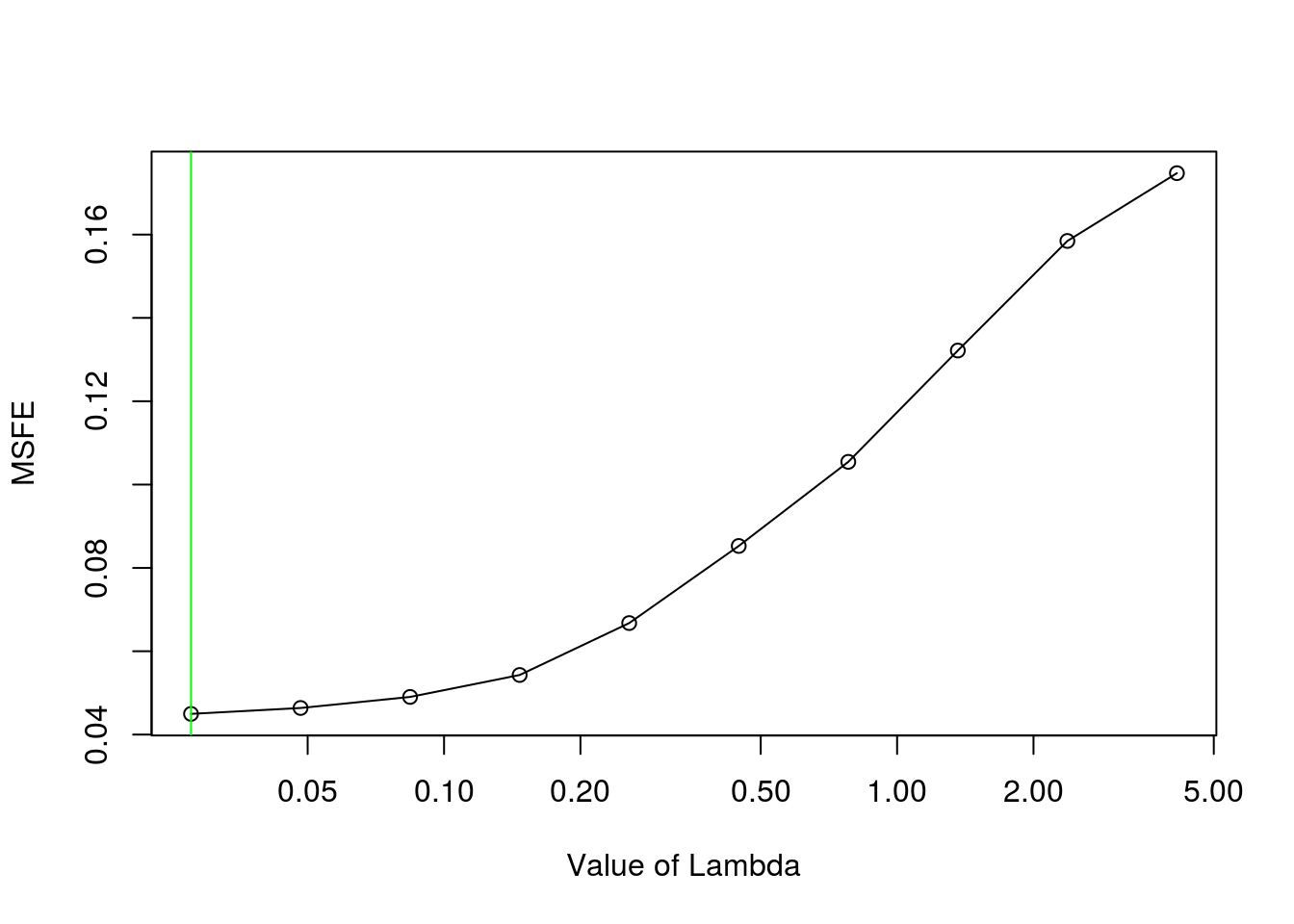Generally, you want this graph to have a parabolic shape with the optimal value in one of the middle indices. In this scenario, since the slope of the line is very flat, it is likely that increasing the depth of the grid (i.e. the first parameter of $${\tt gran}$$ in $$\tt{constructModel}$$) would not substantially improve forecasts. It is not recommended to make the depth too large as it substantially increases computation time.


mod2<-constructModel(Y,p=4,"Basic",gran=c(5,10),h=1,cv="Rolling",verbose=FALSE,IC=FALSE)
res2=cv.BigVAR(mod2)
plot(res2)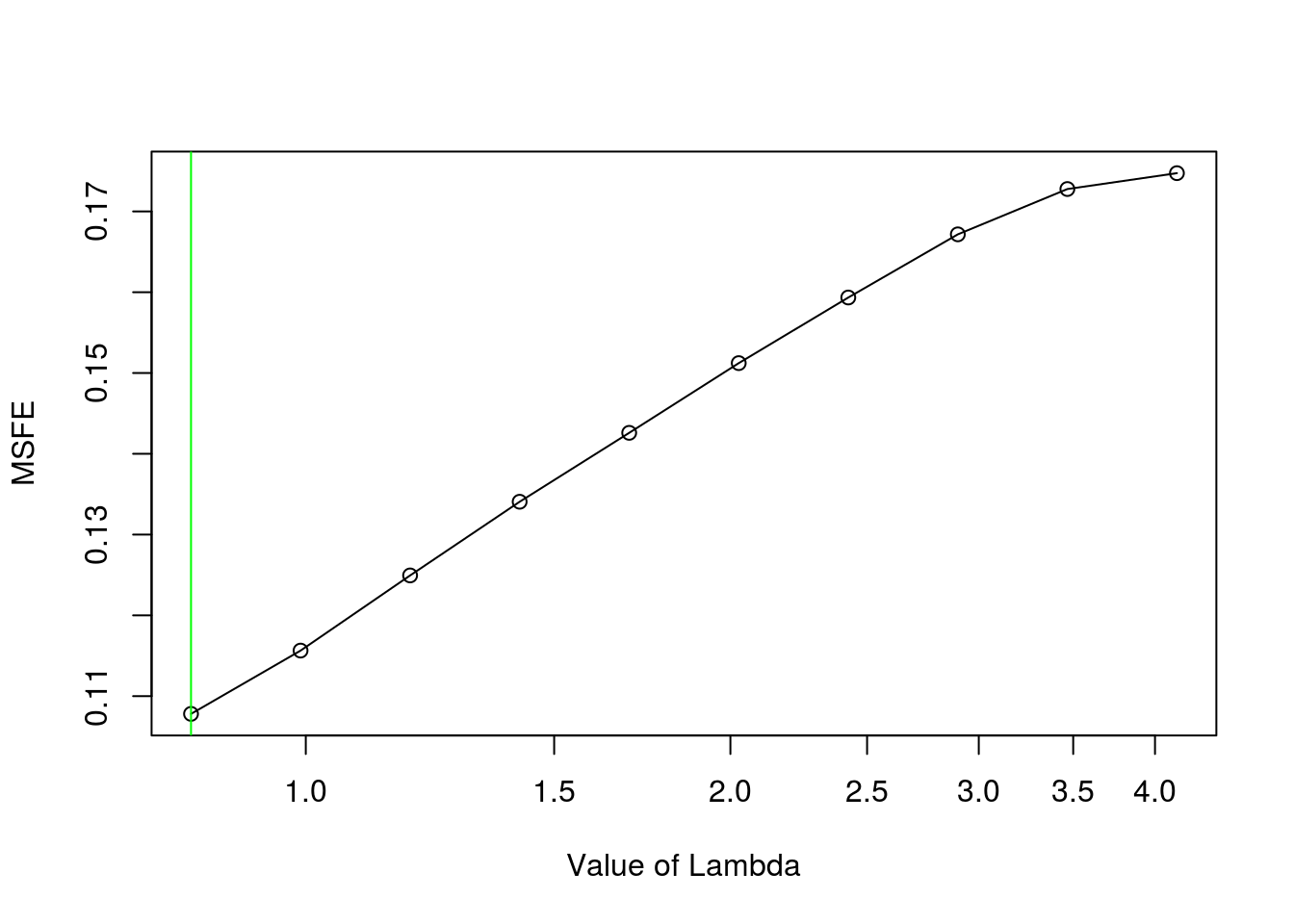However, since the slope of the line in this case is quite steep, it is likely that forecasts will be improved by increasing the depth.


mod3<-constructModel(Y,p=4,"Basic",gran=c(500,10),h=1,cv="Rolling",verbose=FALSE,IC=FALSE)
res3=cv.BigVAR(mod3)
plot(res3)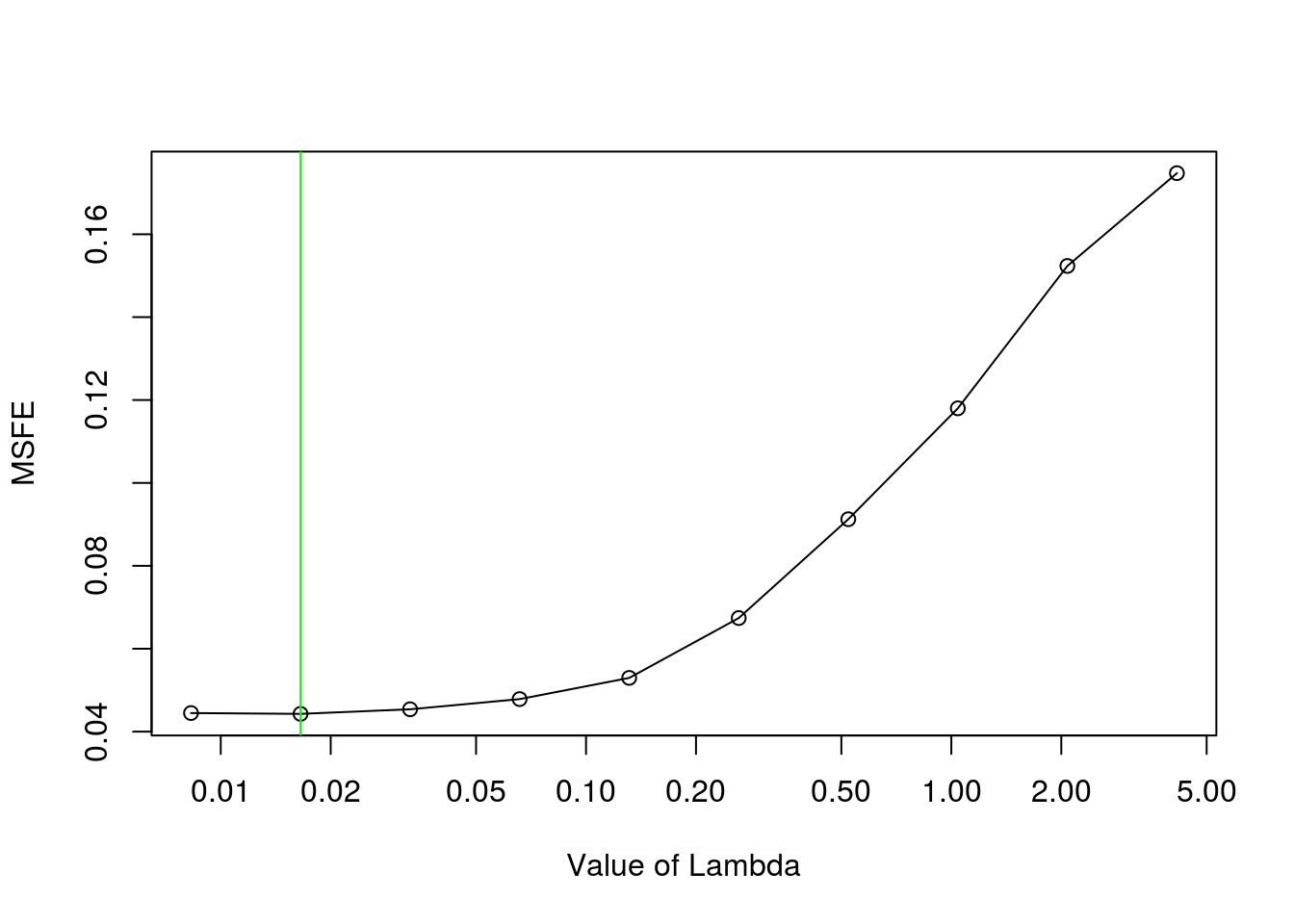As evidenced above, this plot does not always take on a parabolic shape. On occasion, when the grid is very deep, it will start to level off. In this scenario, it is best to decrease the depth of the grid.

## Prediction

We can also view the sparsity pattern of the final estimated coefficient matrix with $${\tt \verb|SparsityPlot.BigVAR.results|}$$

SparsityPlot.BigVAR.results(results)